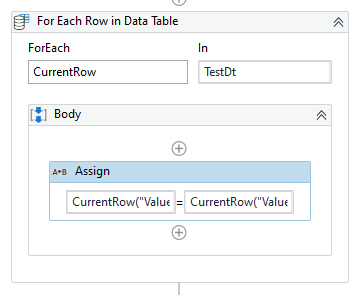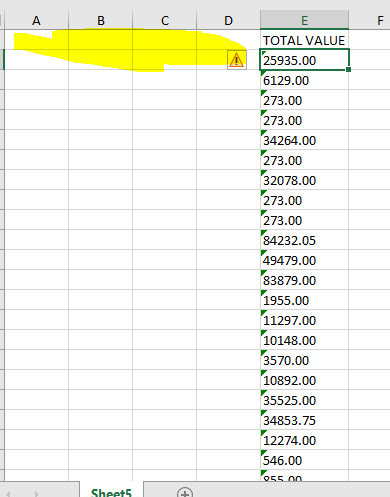# How to remove empty spaces in datatable

How to remove empty spaces in datatable. In datatable some fields are coming along with spaces can anyone please suggest how can i fix this issue.Im attaching sample excel .
Exceldata.xlsx (8.4 KB)

Hey!

Use filter data table activity and mention the column name

Example:

Remove

0 = " "
AND
0=“”

Store it in a new data table thats it

Regards,
NaNi

Hey @Chippy_Kolot,

You can clone the structure of input datatable and load the data from input to cloned datatable by removing the spaces.

``````inputDTClone = inputDt.Clone

(From row In inputDt.AsEnumerable
row("col1").ToString.Trim
.
.
.
row("lastCol").ToString.Trim
}, True)).CopyToDataTable
``````

Thanks,
Sanjit

I was unable to find any empty spaces in the excel sheet provided, Could you maybe point out as to what exactly you want to remove from the Data ?

Also, Maybe the below post contains the solution you’re looking for :

Hello @Chippy_Kolot ,

As the data in input file is very less use for loop and trim the data as shown below :(Assign : CurrentRow(“Value”).ToString = CurrentRow(“Value”).ToString.Trim)

Regards,
Rohith

EXCELDATA1.xlsx (8.9 KB)
This is the excel here I’m facing space issue I’m not able to perform calculations, can you please suggest to remove the space.

Are you referring to the Empty Columns before the `TOTAL VALUE` Column ?No, I m mentioning about Total_Value column.

@Chippy_Kolot , Could you let us know what calculation issues/Errors you faced when using this data ?

Also, let us know what was implemented for this operation.

I have to find the sum of Total Value column, while doing it manually itself not getting the actual value.=SUM(E2:E31)

(From r in DT.Asenumerable
Ler r = r.itemarray.Select(Function(v) v.Tostring.Trim).Toarray

The Numbers in Excel are in String/Text format, hence, it is not able to compute the right value.

You could first change the value to number format and then try using the formula.

For Calculating Sum of the Column in the Datatable manner you could use the below :

``````DT.AsEnumerable.Sum(Function(x)CDbl(x("TOTAL VALUE").ToString))
``````

Let us know if you get an error when using the above expression.

1 Like

Your data is in text format. You can extract data from excel file to data table, convert the required column data into numbers and calculate the total sum. If you need to add the sum as text, convert it back to string. I think the most neat way to do it is using ‘for each row loop’ and use assign activity to cast data type and adding values. You don’t need to trim spaces.

Regards,
Sabahat

This topic was automatically closed 3 days after the last reply. New replies are no longer allowed.